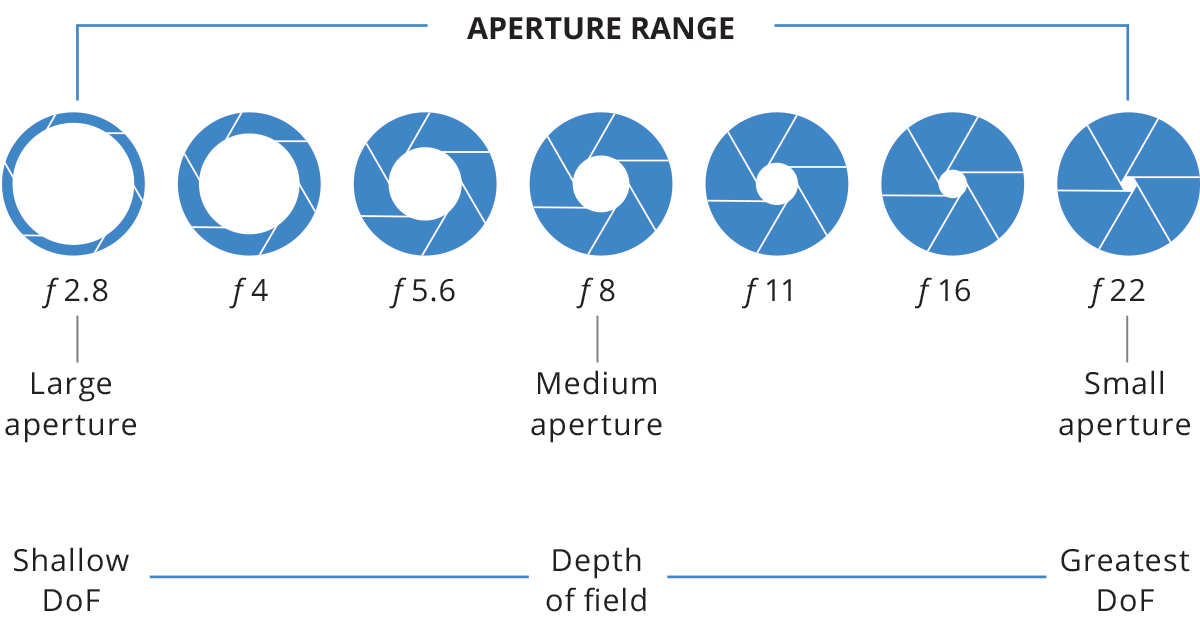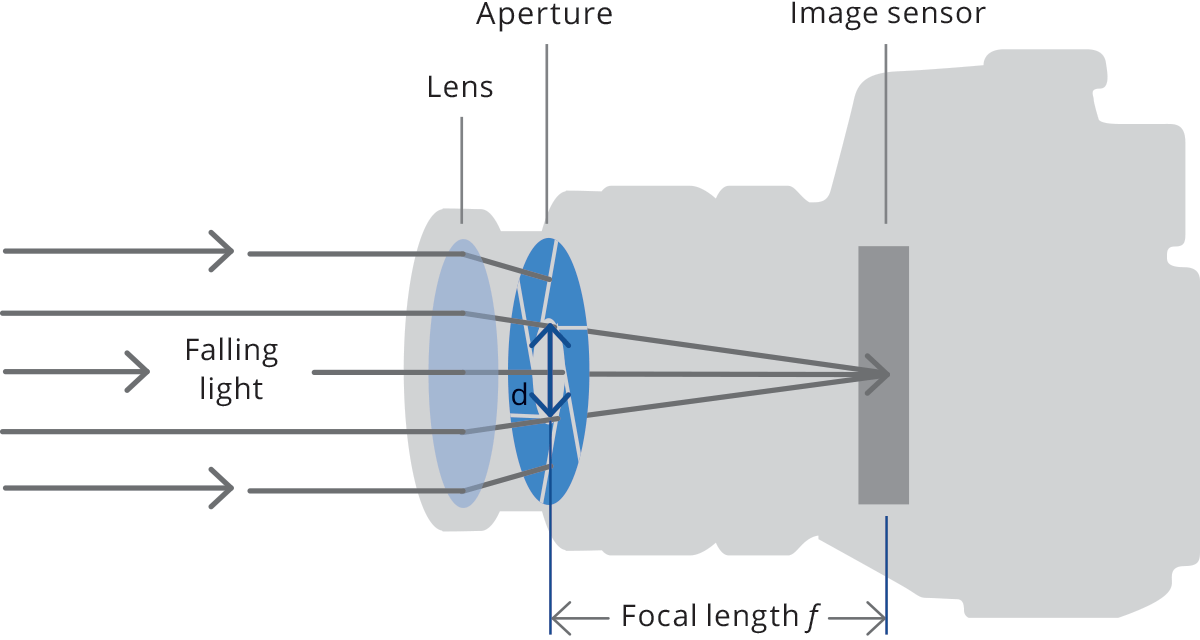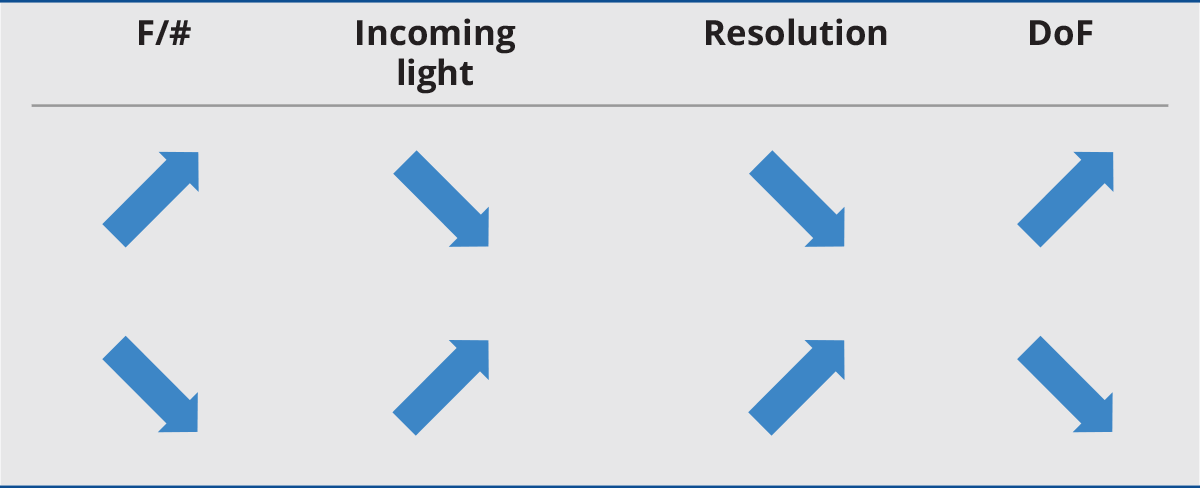# F/# and depth of field

Every optical system is characterized by an aperture stop, that determines the amount of light that passes through it. For a given aperture diameter d and focal length f we can calculate the optics F-number:

F//# = f / d

Typical F-numbers are F/1.0, F/1.4, F/2, F/2.8, F/4, F/5.6, F/8, F/11, F/16, F/22, etc. Every increment in the F-number (smaller aperture) reduces incoming light by a factor of 2. The given definition of F-number applies to fixed focal length lenses where the object is located ‘at infinity’ (i.e. a distance much greater than its focal length). For macro and telecentric lenses where objects are at a closer distance, instead, the working F/# (wF/#)is used. This is defined as:

WF//# = (1 + M) * F//#The F-number affects the optics depth of field (DoF), that is the range between the nearest and farthest location where an object is acceptably in focus. Depth of field is quite a misleading concept because physically there is one and only one plane in object space that is conjugate to the sensor plane. However, being mindful of diffraction, aberration and pixel size, we can define an “acceptable focusing distance” from the image conjugate plane, based on subjective criteria. For example, for a given lens, the acceptable focusing distance for a precision gauging application requiring a very sharp image is smaller than for a coarse visual inspection application.A common F-number value is F/8 since smaller apertures could give rise to diffraction limitations, while lenses with larger apertures are more affected by optical aberrations and distortion. A rough estimate of the field depth of telecentric and macro lenses (or fixed focal length lenses used in macro configuration) is given by the following formula:

DoF [mm] = WF//# * p[µm] * k // M^2

where p is the sensor pixel size (in microns), M is the lens magnification and k is a dimensionless parameter that depends on the application (reasonable values are 0.008 for measurement applications and 0.015 for defect inspection). For example, taking p = 5.5 µm and k = 0.015, a lens with 0.25X mag and WF/# = 8 has an approximate DoF = 10.5 mm.Next →